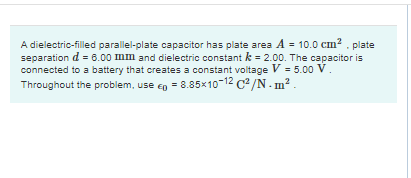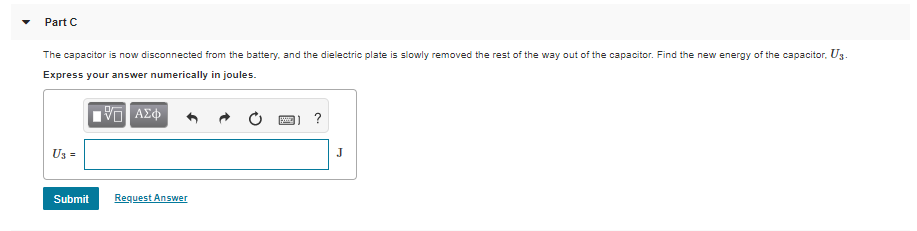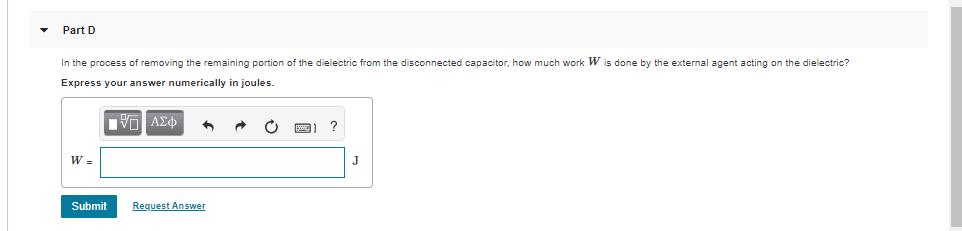# (Solved): NEED HELP DO NOT POST THE FOLLOWING ANSWERS IT IS WRONG AND WILL RESULT IN A DOWN VOTE. DO NOT POST ...NEED HELP DO NOT POST THE FOLLOWING ANSWERS IT IS WRONG AND WILL RESULT IN A DOWN VOTE. DO NOT POST 1.843X10^-11 AND THE SAME FOR THE NEXT PART

A dielectric-filled parallel-plate capacitor has plate area A = 10.0 cm . plate separation d = 8.00 mm and dielectric constant k = 2.00. The capacitor is connected to a battery that creates a constant voltage V = 5.00 V. Throughout the problem, use Ep = 8.85x10-12 C2/N m ? Part C The capacitor is now disconnected from the battery, and the dielectric plate is slowly removed the rest of the way out of the capacitor. Find the new energy of the capacitor. Uz Express your answer numerically in joules. IVO AO OE? Submit Request Answer Part D In the process of removing the remaining portion of the dielectric from the disconnected capacitor, how much work W is done by the external agent acting on the dielectric? Express your answer numerically in joules. VADO W Submit Request Answer

We have an Answer from Expert